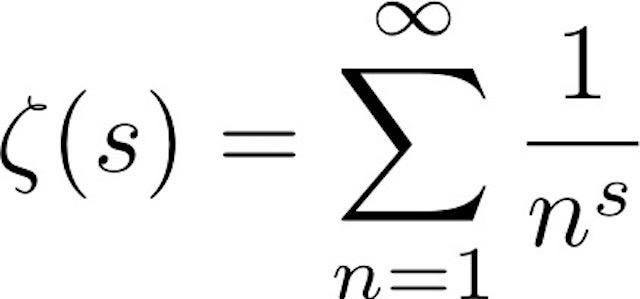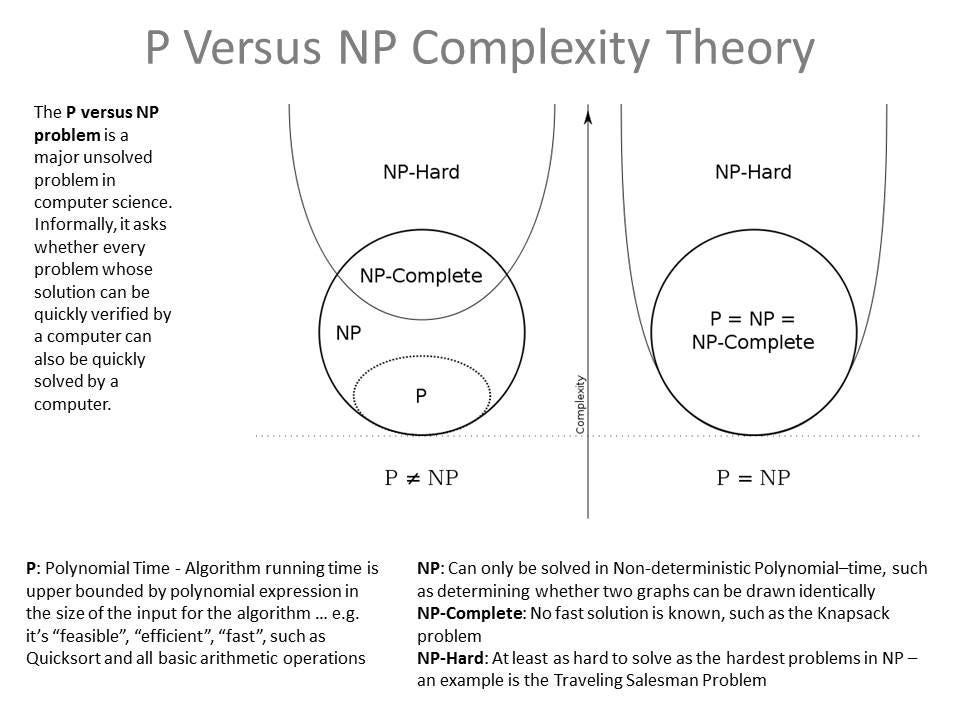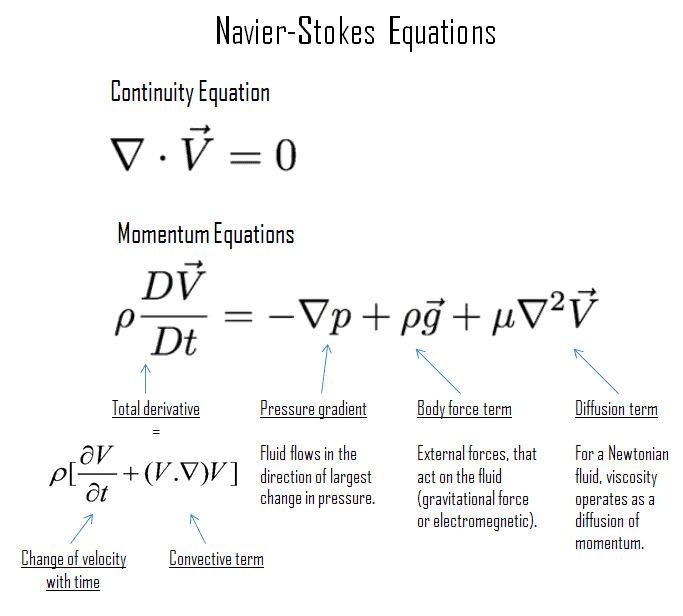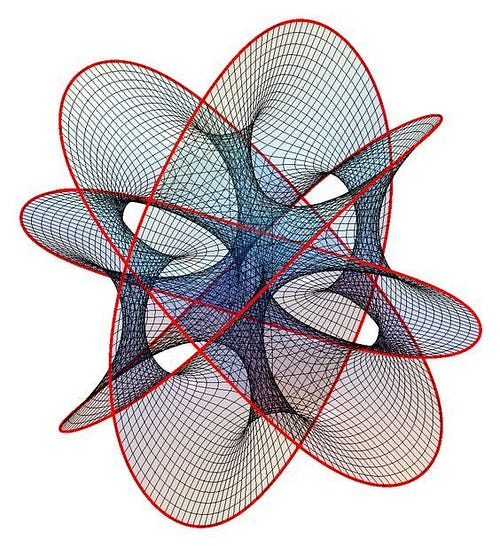* indicates a required field• phone number format invalid• email format invalidProblems detected, please review the form.
Privacy - Terms

Nov 15, 2021

The ‘Million Dollar Problems’ In Mathematics

Nov 2 · 5 min read

You solve one of these problems, you win 1 Million dollars.

In year 2000, the Clay Institute of Mathematics at Oxford, issued a list of seven mathematical problems that are considered the hardest to solve. They are known as “The Millennium Prize Problems”. The individual who will solve any of the problems will be awarded US\$1 million prize by the institute. Some of them are even centuries old and their solutions might result in unlocking many important discoveries in physics, computers and many other fields of science.

Here are the six (out of seven) millennium prize problems in mathematics stated by the Institute.

1) Riemann Hypothesis

The Riemann zeta function ζ(s) is a fn whose argument ‘s’ may be any complex number other than 1, and whose values are also complex. The hypothesis is that all non-trivial zeroes of the analytical continuation of the zeta function have a real part of 1/2.

Formulated in Riemann’s 1859 paper and The official statement of the problem was given by Enrico Bombieri. A proof or disproof of this would have far-reaching implications in number theory, especially for the distribution of prime numbers.2. P vs NP ProblemThe problem of determining whether P = NP is the most important open problem in theoretical computer science. The class of problems in P is the set of problems for which a solution can be found in polynomial time.
The class of problems in NP is the set of problems for which a solution can be verified in polynomial time. So the question is: If it is easy to check that a solution to a problem is correct, is it also easy to solve the problem?

3. Navier–Stokes Equation

The Navier-Stokes equations are partial differential equations modeling the motion of liquids or gases. The problem is, for the three-dimensional system of equations, and given some initial conditions, it not yet proven that smooth solutions always exist.These are important equations in fluid mechanics. The problem, restricted to the case of an incompressible fluid, is to prove either that smooth, globally defined solutions exist that meet certain conditions, or that they do not always exist and the equations break down.
The equations are named after French engineer and physicist Claude-Louis Navier and Irish English physicist and mathematician Sir George Stokes.

4. Yang-Mills Existence and Mass Gap

In quantum field theory, the mass gap is the difference in energy between the vacuum and the next lowest energy state. Experiments suggest the existence of a “mass gap” in the solution to the quantum versions of the Yang-Mills equations.

Quantum Yang–Mills theory is a generalization of the Maxwell theory of electromagnetism where the chromo-electromagnetic field itself carries charge. It has solutions which travel at the speed of light so that its quantum version should describe gluons.
However, the color confinement process permits only bound states of gluons, forming massive particles. This is the mass gap. The problem is to establish rigorously the existence of the quantum Yang–Mills theory and a mass gap.
The theory is named after theoretical physicists Dr. Chen Ning Yang and Dr. Robert Laurence Mills. Yang-Mills theory has been instrumental in the Standard Model of Particle Physics. It provides a framework for explaining Electromagnetic and nuclear forces and classifying subatomic particles.C. N. Yang and Robert Mills at Stony Brook in 1999.

5. Hodge Conjecture

Algebraic geometry deals with the higher-dimensional analogues of the ‘classical curves’ when one considers systems of multiple equations, equations with more variables, and equations over the complex number plane, rather than the real numbers.These difficult-to-imagine shapes can be made more tractable through complicated computational tools. The Hodge conjecture suggests that certain types of geometric structures have a useful algebraic counterpart that can be used to better study and classify these shapes.

The Hodge conjecture is known in certain special cases, e.g., when the solution set has dimension less than four. But in dimension four it is unknown. The conjecture was formulated by Hodge in 1950.

6. Birch-Swinnerton-Dyer Conjecture

The Birch-Swinnerton-Dyer conjecture concerns the rational points (points with all coordinates rational numbers) on elliptic curves. Elliptic curves, defined by cubic equations in two variables, are fundamental mathematical objects. They arise in many areas including Wiles’ proof of the Fermat Conjecture, factorization of numbers into primes, and cryptography. It is named after mathematicians Bryan John Birch and Peter Swinnerton-Dyer, who developed the conjecture during the first half of the 1960s.Bryan John Birch and Peter Swinnerton-Dyer outside the Parish church in Solihull, July 1, 1961.

The only problem solved so far is the Poincaré conjecture. A proof of this conjecture was given by Grigori Perelman in 2003. Perelman’s solution was based on Richard Hamilton’s theory of Ricci flow. You can check out my article on the problem here: